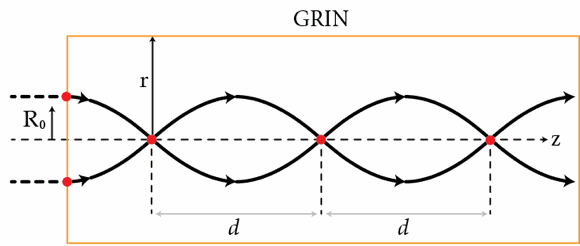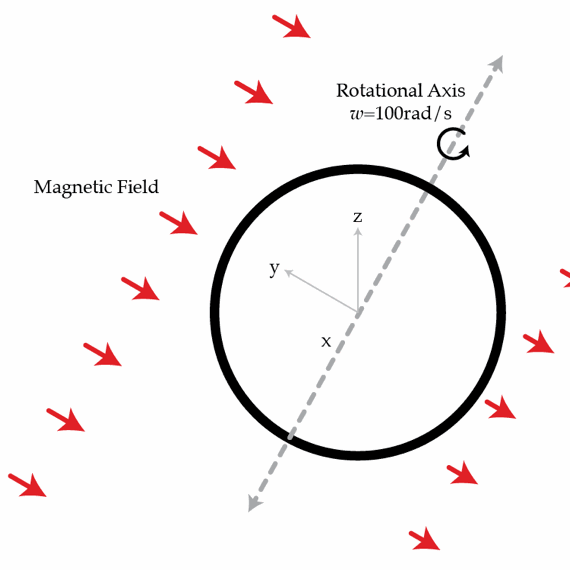Electricity and Magnetism

# E+M Warmups: Level 5 Challenges

Consider a cylindrical optical fiber with a core that has a graded index (GRIN) of refraction given by $n(r)=n_{0}\sqrt{1- (g r)^2}$ where $r$ is the distance to from the optical axis of the fiber. Show that this profile results in continual refocusing of paraxial rays as shown in the figure below. If $g=0.33~\mbox{mm}^{-1}$, determine the distance $d$ in millimeters between two consecutive focal points.A thin ring with resistance $R=10~\Omega$ and self inductance $L=0.2~\mbox{H}$ rotates with constant angular speed $\omega=100~\mbox{rad/s}$ in an external uniform magnetic field perpendicular to the rotation axis. As a result, the magnetic flux created by the external field varies with time as $\Phi(t)=\Phi_{0}\cos(\omega t) \quad \textrm{where} \quad \Phi_{0}=0.1~\mbox{Wb} .$ Because of the resistance of the ring, energy is being continuously dissipated in the system. What average power $\bar{P}$ in Watts must develop the external forces to keep the ring rotating at constant angular speed $\omega$ (on average).Consider two balls of equal radii and masses but opposite charges, distributed uniformly over their volumes. Initially, the balls are at rest and far away from one another. Due to the Coulomb attraction, the balls start moving towards each other. The balls can be treated as charged clouds, that is to say, they can interpenetrate without friction. During the interaction, the maximum speed achieved by the balls is $v_{max}=10~\text{m}/\text{s}$. What is the magnitude of their maximum acceleration in meters per second squared? The radius of the balls is $R=1~\text{m}$.

An electron is accelerated by a potential difference of $U_e= 1~\mbox{mV}$. It then enters a region with an inhomogeneous magnetic field $\vec{B}(x,y,z)$ generated by a system of coils carrying currents $I_{1}, I_{2}\ldots$.

We then perform a similar experiment with a proton. We first reverse the current in all the coils generating the magnetic field. We then accelerate the proton with a potential difference of $U_p$ and it enters the region with the magnetic field. What must $U_p$ be in Volts so that the trajectory of the proton is the same as that of the electron? Don't forget any sign changes!

Hint: Compute $\frac{d}{ds} \frac{\vec{v}}{v},$ where $s$ is the arc length along the trajectory:

$s=\int_0^t v(t') dt'$.

Details and assumptions

The proton to electron mass ratio is approximately $6 \pi^{5}$.

In 1-d Newtonian mechanics the energy as a function of momentum for a particle is given by $E=\frac{1}{2}mv^2$ or, in terms of momentum, $E=p^2/(2m)$. In Einstein's theory of special relativity there is still energy and momentum, and they are still conserved, but the relationship between the two is different. In empty space, the energy of a particle as a function of its momentum $\vec{p}$ and mass $m$ is given by $E^2=c^2 \vec{p} \cdot \vec{p}+m^2c^4$ where $c$ is the speed of light. The photon, the "particle" of light, has no mass - its energy satisfies $E=c|\vec{p}|$ in empty space. In a medium like water this can change - photons travel slower in a medium and their energy-momentum relation becomes $E=c_m |\vec{p}|$, where $c_m$ is the speed of light in the medium. Our question is the following: A very high energy proton with $E_{prot}^2=c^2 \vec{p} \cdot \vec{p}+m_{prot}^2c^4$ enters a tank of water. What is the minimum magnitude of the proton's momentum, i.e. $|\vec{p}|$, in kg m/s, such that the proton can emit a photon with non-zero momentum and still conserve energy and momentum?

Details and assumptions

• The speed of light in empty space is $3 \times 10^8~\mbox{m/s}$.
• The mass of the proton is $1.67 \times 10^{-27}~\mbox{kg}$.
• The speed of light in water is $2.3 \times 10^8~\mbox{m/s}$.
• When photon emission begins to occur the initial proton, the final proton, and the emitted photon all travel in the same direction.
×

Problem Loading...

Note Loading...

Set Loading...